• 2021-08-05 17:35:22
import numpy as np
import matplotlib.pyplot as plt
from sklearn import datasets
from sklearn.linear_model import LinearRegression
from sklearn.model_selection import train_test_split
from sklearn.metrics import mean_squared_error

class Linear_Regression():
def __init__(self):
self.lr = 0.01
self.num_iter = 500
self.w = None

def fit(self,X,y):
X = np.hstack((X,np.ones(X.shape).reshape(-1,1)))
print(X.shape)
self.w = np.linalg.pinv(X.T @ X) @ X.T @ y

def predict(self,X):
X = np.hstack((X,np.ones(X.shape).reshape(-1,1)))
y_pred = X @ self.w
return y_pred

if __name__ == '__main__':
# X = np.mat(X)
# y = np.mat(y).reshape(-1,1)
print(X.shape)
print(y.shape)
model = LinearRegression()
LR_MSE_list = []
ND_MSE_list = []
for _ in range(10):
X_train, X_test, y_train, y_test = train_test_split(X,y,test_size=0.3)
model = Linear_Regression()
model.fit(X=X_train, y=y_train)
y_pred = model.predict(X=X_test)

print(mean_squared_error(y_true=y_test, y_pred=y_pred))

model_1 = LinearRegression()
model_1.fit(X=X_train, y=y_train)
y_pred = model_1.predict(X=X_test)
print("diaobao::",mean_squared_error(y_true=y_test, y_pred=y_pred))

break

更多相关内容
• 多元回归-最小二乘法-残差分析笔记 一.多元线性回归模型的假设 我们需要进行以下六个假设，这些假设是经典的多元线性回归模型有效的前提： 1、因变量Y和自变量X1，X2，…，Xk之间的关系是线性的。 2、自变量（X1，X2...
• 用matlab编写实现最小二乘法多元线性拟合，可以得到最终拟合方程，并画出预测的回归系数直方图
• 多元回归的一般式 y^(θ,x)=θ0+θ1x1+…+θpxp\hat{y}(\theta, x)=\theta_{0}+\theta_{1} x_{1}+\ldots+\theta_{p} x_{p}y^​(θ,x)=θ0​+θ1​x1​+…+θp​xp​ 其中： y^\hat{y}y^​:预测值 θ0,θ1,…θp\...

## 线性回归的原理

• 多元回归的一般式

y ^ ( θ , x ) = θ 0 + θ 1 x 1 + … + θ p x p \hat{y}(\theta, x)=\theta_{0}+\theta_{1} x_{1}+\ldots+\theta_{p} x_{p}

其中：
y ^ \hat{y} :预测值

θ 0 , θ 1 , … θ p \theta_{0}, \theta_{1}, \ldots \theta_{p} :参数

x 0 , x 1 , … x p x_{0}, x_{1}, \ldots x_{p} :自变量

• 方程的拟合思路

## 最小二乘法

• 通过求导求极值解法

下面通过一元线性回归模型做推导：

样本回归模型：

y ^ i = β ^ 0 + β ^ 1 x i \hat{y}_{i}=\hat{\beta}_{0}+\hat{\beta}_{1} x_{i}

残差平方和(损失函数):

Q = ∑ i = 1 n e i 2 = ∑ i = 1 n ( y i − y ^ i ) 2 = ∑ i = 1 n ( y i − β ^ 0 − β ^ 1 x i ) 2 Q=\sum_{i=1}^{n} e_{i}^{2}=\sum_{i=1}^{n}\left(y_{i}-\hat{y}_{i}\right)^{2}=\sum_{i=1}^{n}\left(y_{i}-\hat{\beta}_{0}-\hat{\beta}_{1} x_{i}\right)^{2}

通过使得Q最小时的参数确定直线，即把它们看作是Q的函数，就变成了一个求极值的问题，可以通过求导数得到。求Q对两个待估参数的偏导数

{ ∂ Q ∂ β ^ 0 = 2 ∑ i = 1 n ( y i − β ^ 0 − β ^ 1 x i ) ( − 1 ) = 0 ∂ Q ∂ β ^ 1 = 2 ∑ i = 1 n ( y i − β ^ 0 − β ^ 1 x i ) ( − x i ) = 0 \left\{\begin{aligned} \frac{\partial Q}{\partial \hat{\beta}_{0}} &=2 \sum_{i=1}^{n}\left(y_{i}-\hat{\beta}_{0}-\hat{\beta}_{1} x_{i}\right)(-1)=0 \\ \frac{\partial Q}{\partial \hat{\beta}_{1}} &=2 \sum_{i=1}^{n}\left(y_{i}-\hat{\beta}_{0}-\hat{\beta}_{1} x_{i}\right)\left(-x_{i}\right)=0 \end{aligned}\right.

解得：

β ^ 1 = n ∑ x i y i − ∑ x i ∑ y i n ∑ x i 2 − ( ∑ x i ) 2 \hat{\beta}_{1}=\frac{n \sum x_{i} y_{i}-\sum x_{i} \sum y_{i}}{n \sum x_{i}^{2}-\left(\sum x_{i}\right)^{2}}

β ^ 0 = ∑ x i 2 ∑ y i − ∑ x i ∑ x i y i n ∑ x i 2 − ( ∑ x i ) 2 \hat{\beta}_{0}=\frac{\sum x_{i}^{2} \sum y_{i}-\sum x_{i} \sum x_{i} y_{i}}{n \sum x_{i}^{2}-\left(\sum x_{i}\right)^{2}}

其中：

β ^ 0 = y ˉ − β ^ 1 x ˉ \hat{\beta}_{0}=\bar{y}-\hat{\beta}_1 \bar{x}

β ^ 1 = ∑ i = 1 n x i y i − n x y ‾ ∑ i = 1 n x i 2 − n x ˉ 2 \hat{\beta}_{1}=\frac{\sum_{i=1}^{n} x_{i} y_{i}-n \overline{x y}}{\sum_{i=1}^{n} x_{i}^2-n \bar{x}^{2}}

• 通过矩阵求解

定义：
h θ ( x 1 , x 2 , … x n − 1 ) = θ 0 + θ 1 x 1 + … + θ n x n − 1 h_{\theta}\left(x_{1}, x_{2}, \ldots x_{n-1}\right)=\theta_{0}+\theta_{1} x_{1}+\ldots+\theta_{n} x_{n-1}
现在有m个样本，每个样本有n−1维特征将所有样本点代入模型中得：

h 1 = θ 0 + θ 1 x 1 , 1 + θ 2 x 1 , 2 + … + θ n − 1 x 1 , n − 1 h 2 = θ 0 + θ 1 x 2 , 1 + θ 2 x 2 , 2 + … + θ n − 1 x 2 , n − 1 ⋮ h m = θ 0 + θ 1 x m , 1 + θ 2 x m , 2 + … + θ n − 1 x m , n − 1 \begin{array}{l} h_{1}=\theta_{0}+\theta_{1} x_{1,1}+\theta_{2} x_{1,2}+\ldots+\theta_{n-1} x_{1, n-1} \\ h_{2}=\theta_{0}+\theta_{1} x_{2,1}+\theta_{2} x_{2,2}+\ldots+\theta_{n-1} x_{2, n-1} \\ \vdots \\ h_{m}=\theta_{0}+\theta_{1} x_{m, 1}+\theta_{2} x_{m, 2}+\ldots+\theta_{n-1} x_{m, n-1} \end{array}

为方便用矩阵表示令 x 0 = 1 x_0 = 1 于是上述方程可以用矩阵表示为：

h = X θ \mathbf{h}=\mathbf{X} \theta
其中，h为m x 1的向量, 代表模型的理论值，θ 为n x 1的向量，X为 m x n维的矩阵，m代表样本的个数,n代表样本的特征数，于是目标损失函数用矩阵表示为：

J ( θ ) = ∥ h − Y ∥ 2 = ∥ X θ − Y ∥ 2 = ( X θ − Y ) T ( X θ − Y ) J(\theta)=\|\mathbf{h}-\mathbf{Y}\|^{2}=\|\mathbf{X} \theta-\mathbf{Y}\|^{2}=(\mathbf{X} \theta-\mathbf{Y})^{T}(\mathbf{X} \theta-\mathbf{Y})

关于矩阵的求导补充以下知识点：

∂ x T a ∂ x = ∂ a T x ∂ x = a ∂ x T A x ∂ x = A x + A T x \begin{aligned} \frac{\partial x^{T} a}{\partial x} &=\frac{\partial a^{T} x}{\partial x}=a \\ \frac{\partial x^{T} A x}{\partial x} &=A x+A^{T} x \end{aligned}
又由于如果矩阵A是对称的：

A x + A T x = 2 A x A x+A^{T} x=2 A x
对目标函数化简：

J ( θ ) = θ T X T X θ − θ T X T Y − Y T X θ + Y T Y J(\theta)=\theta^{T} X^{T} X \theta-\theta^{T} X^{T} Y-Y^{T} X \theta+Y^{T} Y
则θ最小值：

θ ^ = argmin ⁡ θ L ( θ ) ⟶ ∂ ∂ θ L ( θ ) = 0 ⟶ 2 X T X θ ^ − 2 X T Y = 0 ⟶ θ ^ = ( X T X ) − 1 X T Y = X + Y \begin{aligned} \hat{\theta}=\underset{\theta}{\operatorname{argmin}} L(\theta) & \longrightarrow \frac{\partial}{\partial \theta} L(\theta)=0 \\ & \longrightarrow 2 X^{T} X \hat{\theta}-2 X^{T} Y=0 \\ & \longrightarrow \hat{\theta}=\left(X^{T} X\right)^{-1} X^{T} Y=X^{+} Y \end{aligned}
这个式子中 ( X T X ) − 1 X T \left(X^{T} X\right)^{-1} X^{T} 又被称为伪逆写作 X + X^{+} ,对于行满秩或者列满秩的X ，可以直接求解。

• 几何意义法求解

求解 b 在A的列向量空间中的投影。

在几何上，最小二乘法相当于模型（这里就是直线）和试验值的距离的平方求和，假设我们的试验样本张成一个 维空间（满秩的情况）模型写成 f ( w ) = X β f(w)=X \beta 而最小二乘法就是说希望 Y 和这个模型距离越小越好，于是它们的差应该与这个张成的空间垂直：

X T ⋅ ( Y − X β ) = 0 ⟶ β = ( X T X ) − 1 X T Y X^{T} \cdot(Y-X \beta)=0 \longrightarrow \beta=\left(X^{T} X\right)^{-1} X^{T} Y

## python实现最小二乘法（通过矩阵求解）

class LinearRegression:
'''使用Python实现的线性回归.（最小二乘法）'''
def fit(self,X,y):
'''根据提提供的训练数据X，对模型进行训练。

Parameters
------
X ：类数组类型。形状：[样本数量，特征数量]
特征矩阵，用来对模型进行训练

y = 类数组类型，形状：[样本数量]

'''
# 如果X是数组对象的一部分，而不是完整的对象数据（eg:切片）则无法完成矩阵的转换
X = np.asmatrix(X.copy())  #这里创建X的拷贝对象，避免转换成矩阵时候失败
# y 为一维结构（行向量或列向量）没有限制，可以不用拷贝
# 注意：现在要进行矩阵的运算，需要是二维结构，通过reshape方法进行转换
y = np.asmatrix(y).reshape(-1,1)    #    (-1,1)意思：把数组转换为一列，依据数据自定义行的矩阵
# 通过最小二乘公式，求解出最佳的权重值
self.w_ = (X.T * X ).I * X.T * y
def predict(self,X):
'''根据参数传递的样本X，delattr样本数据进行预测。

Parameters
------
X：类数组类型。形状[样本数量，特征数量]
待预测的样本特征（属性）

Returns
------
result:数组类型
预测的结果
'''
# 将X转换为矩阵 注意：需要对X进行拷贝
X = np.asmatrix(X.copy())
result = X * self.w_
# 将矩阵转换为ndarray数组 ，进行扁平化处理 ，然后返回结果
return np.array(result).ravel()

展开全文机器学习 python
• 采用线性回归的方式，Y=bx+a,求出系数 #include#includeintmain(){//floatx={0.0,1.0,2.0,3.0,4.0,5.0};//floaty={1.1,1.9,3.1,3.9,4.4,4.9};/*floatx={0.0,1.0,2.0,3.0,4.0,5.0};floaty={0.0,1.0,2.0,...

采用线性回归的方式，Y=bx+a,求出系数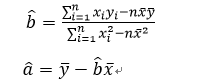#include

#include

int main()

{

//float x={0.0,1.0,2.0,3.0,4.0,5.0};

//float y={1.1,1.9,3.1,3.9,4.4,4.9};

/*float x={0.0,1.0,2.0,3.0,4.0,5.0};

float y={0.0,1.0,2.0,3.0,4.0,5.0};*/

float x={0.0,1.0,2.0,3.0,4.0,5.0};

float y={1.0,1.0,1.0,1.0,1.0,1.0};

float a,b,mxy,xx,yy,x2,x22;

int i;

a=b=mxy=xx=yy=x2=x22=0.0;

for(i=0;i<6;i++)

{

mxy=6.0*x[i]*y[i]+mxy;

xx=1.0*x[i]+xx;

yy=1.0*y[i]+yy;

x2=1.0*x[i]*x[i]*6.0+x2;

x22=1.0*x[i]+x22;

}

b=1.0*(mxy-xx*yy)/(x2-x22*x22);

a=1.0*yy/6.0-b*xx/6.0;

printf("Y=%0.2fx+%0.2f\n",b,a);

system("pause");

return 0;

}

Y=0.00x+1.00

展开全文• 多元线性回归 最小二乘法实现   线性回归包括一元线性回归多元线性回归，一元的是只有一个x和一个y。多元的是指有多个x和一个y。     对于平面中的这n个点，可以使用无数条曲线来拟合。要求样本...

为什么会用纯Python来实现呢？这种陶（hao）冶（wu）情（yong）操（chu）的做法肯定也不是我自己闲着蛋疼搞着玩的。。。  明明有更好的科学计算库不用，非要纯手写正面硬刚，除了老师用挂科作为威胁还能有谁？

下面是一大纯手打的堆理推导... 写的逻辑有些混乱，后续有时间再慢慢整理 觉得麻烦的小伙伴就不用看了，反正我代码里也写的有注释，代码稍后传上github后更新连接

---------------------------------------------------------------------------------------------------------------

多元线性回归 最小二乘法实现

线性回归包括一元线性回归和多元线性回归，一元的是只有一个x和一个y。多元的是指有多个x和一个y。

对于平面中的这n个点，可以使用无数条曲线来拟合。要求样本回归函数尽可能好地拟合这组值。综合起来看，这条直线处于样本数据的中心位置最合理。 选择最佳拟合曲线的标准可以确定为：使总的拟合误差（即总残差）达到最小。有以下三个标准可以选择：

（1）用“残差和最小”确定直线位置是一个途径。但很快发现计算“残差和”存在相互抵消的问题。
（2）用“残差绝对值和最小”确定直线位置也是一个途径。但绝对值的计算比较麻烦。
（3）最小二乘法的原则是以“残差平方和最小”确定直线位置。用最小二乘法除了计算比较方便外，得到的估计量还具有优良特性。这种方法对异常值非常敏感。

样本x为一个多维向量，x=[x1,x2,…xd]

那么权重W也是与X同维度的一个向量，W=[w1,w2,…wd]

所以Y对x的预测可以写为Y=W ∙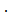x+b(这里为内积的形式)，但通常将b移到W向量里面去，写为W=[b,w1,w2,…wd]，改变后的W用θ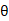表示，θ=[θ0,θ1,θ2,…θd]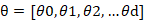因为W的维度变了所以我们也要给x对应的多加一个维度，此时的x为[x0,x1,…xd]，使x0等于1，让其在矩阵相乘时匹配刚才在W中加入的b

现在Y=W ∙x+b 就等价为 Y=θ∙x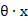在机器学习的矩阵运算当中，有个约定俗称的规定，当说某某向量时都指的是一个列向量，所以θ和x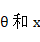都是一个d+1维的列向量[θ0θd]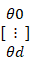、[x0xd]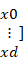，同时也都是一个(d+1)x1维的矩阵

这里的维度就对应方程里面的元数，有几维就是几元

所以这里用大写的X表示有m个样本，X=[x1TxmT⋯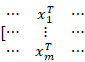]=[x01xd1x0mxdm]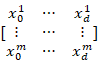，（这里的X是一个矩阵，包含了所有的样本点x1,x2,…xm而每一个样本点都是有相同的多维的， [x1TxmT⋯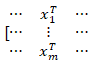]* [θ0θd]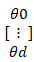= y0ym=Y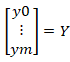，得到一个m*1维的矩阵Y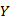，这个矩阵中的每一个参数代表给定的θ参数下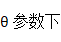对每一个样本xi的预测值yi，Y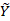是对应样本的标签值，也是一个列向量。

得到Y（预测值）过后，就可以算损失函数了，L=i=1m（yi-yi）2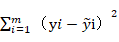y是对应样本的真实值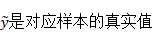L=（y1-y1）2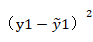+（y2-y2）2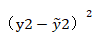+…+（ym-ym）2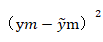=[ y1-y1,,ym-ym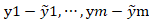][y1-y1ym-ym]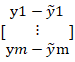=(y1,⋯,ym-[y1,,ym])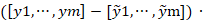[y1-y1ym-ym]=(x∙θ-Y)T ∙  (xθ-Y)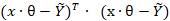因为x、θ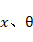Y都是一个m+1维的列向量所以第一项(x∙θ-Y)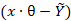的结果也是一个列项量，所以加了一个转置

得到损失函数L=(x∙θ-Y)T (xθ-Y)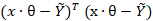，通过改变θ的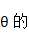值来最小化L

对θ求偏导，∂L∂θ=(θTxT-YT)(xθ-Y)∂θ=θTxTxθ-θTxTY-YTxθ+YTY∂θ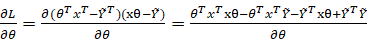，每一项都对θ求偏导然后加起来

∂L∂θ=2xTxθ-xTY-xTY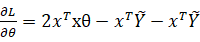，要使损失函数最小，就是偏导为0的点

所以推出xTxθ=xTY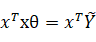，θ=( xTx)-1xTY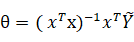，这就要求(xTx)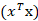是可逆的

最小二乘法与梯度下降法的区别：

实现方法和结果不同：最小二乘法是直接对∆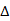求导找出全局最小，是非迭代法。而梯度下降法是一种迭代法，先给定一个β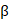，然后向∆下降最快的方向调整β，在若干次迭代之后找到局部最小。梯度下降法的缺点是到最小点的时候收敛速度变慢，并且对初始点的选择极为敏感，其改进大多是在这两方面下功夫。

展开全文• 在数据分析中，线性回归是最简单且最有效的分析方法。举个简单的例子，某商品的利润在售价为2元、5元、10元时分别为4元、10元、20元，我们很容易得出商品的利润与售价的关系符合直线：.在上面这个简单的一元线性回归...python
• 这里写自定义目录标题前言实现原理工具准备1， 线性回归最小二乘参数估计使用python计算最小二乘法的线性回归检验 前言 本次知识来自贾俊平《统计学》第七版，初次学习，如有纰漏，请各位多多包含。诸位之意见笔者会...python
• 1. 最小二乘法损失函数求解推导； 2. sklearn中linear_model.LinearRegression参数介绍+案例 最小二乘法损失函数求解推导 最小二乘法的思路：对损失函数求导，令其为0，求得损失函数最小值时的参数，但前提条件：...python
• 最小二乘法的主要思想是通过确定未知参数θ\thetaθ（通常是一个参数矩阵），来使得真实值和预测值的误差（也称残差）平方和最小，其计算公式为E=∑i=0nei2=∑i=1n(yi−yi^)E=\sum_{i=0}^ne_i^2=\sum_{i=1}^n(y_i-\...机器学习
• 最小二乘法是先将方程自变量与因变量化为系数矩阵X，再求该矩阵的转置矩阵（X1），接着求矩阵X与他的转置矩阵的X1的乘积（X2），然后求X2的逆矩阵。最后整合为系数矩阵W，求解后分别对应截距b、a1、和a2。可见计算一...
• 在常规预测分析过程中如果预测的变量是连续的，最为常用的预测方式为回归分析，具体包括线性、非线性2类，其核心是采用最小二乘法（直线到各点的距离之和最小）对已知的样本数据进行最优拟合，然后通过拟合出的线性...机器学习
• 欢迎关注”生信修炼手册”!线性回归模型是使用最广泛的模型之一，也最经典的回归模型，如下所示x轴表示自变量x的值，y轴表示因变量y的值，图中的蓝色线条就代表它们之间的回归模型，在该模型中，...机器学习 人工智能 数据挖掘
• 【MATLAB实战应用源代码】MATLAB实现最小二乘法函数多元线性回归&多项式拟合.zip
• 普通最小二乘线性回归 一般情况下，线性回归模型假设函数为： hw,b(x)=∑i=1nwixi+b=wTx+b h_{w, b}(x)=\sum_{i=1}^{n} w_{i} x_{i}+b=w^{\mathrm{T}} x+b hw,b​(x)=i=1∑n​wi​xi​+b=wTx+b 其中，w∈Rnw\in \...
• 设随机变量yyy与一般变量x1,x2,...,xpx_{1},x_{2},...,x_{p}x1​,x2​,...,xp​的线性回归模型为： y= y= y= 样本(x,y)(x,y)(x,y)可由y=β0+β1x+εy=\beta _{0}+\beta _{1}x+\varepsilony=β0​+β1​x+ε 表示，...
• 机器学习-线性回归线性回归背景最小二乘法计算线性回归数据的准备把数据转换成数学损失函数根据损失函数计算最终结果总结 最近老师让我学习线性回归编程模型，此篇介绍使用最小二乘法实现单机器线性回归。 线性回归...公式推导 机器学习
• 线性回归模型可以说是最重要的数学模型之一，很多模型都是建立在它的基础之上，可以被称为是“模型之母”。 kNN算法属于分类(Classification)，即label为离散的类别型(categorical variable)，如：颜色类别、手机...机器学习 python
• 方法介绍“最小二乘法”一句话解释：一种数学优化方法，通过最小化误差的平方和来寻找合适的数据拟合函数。 线性模型的最小二乘可以有很多方法来实现，比如直接使用矩阵运算求解析解，sklearn包（参考：用scikit-...
• 最小二乘法”一句话解释：一种数学优化方法，通过最小化误差的平方和来寻找合适的数据拟合函数。 线性模型的最小二乘可以有很多方法来实现，比如直接使用矩阵运算求解析解，sklearn包（参考：用scikit-learn和...
• 最小二乘法多元线性回归（岭回归实现）多元线性回归回归实现一、理论分析二、实现代码第一部分（LinRegression类）第二部分（main，调用类并做对比）三、代码解读及结果分析1.代码解读2.结果分析 采用岭回归的方式...机器学习 python
• 最小二乘法的目标函数可以简单理解成下面这个表达式，其中是理论值，是观测值。 为了简化问题，我们将视为一个一次函数，其表达式为。 已知 求最佳的和，使得上述目标函数取最小值 2. 数学推导 将.matlab 开发语言
• 假设给定d个属性的样本为,线性回归就是想找到一个这d个属性的找到一个预测函数 （1），用线性代数中的向量表示为 （2），我们先随机给w和b赋值，之后利用训练集不断的调整w和b使得准确率不断提高。从上述公式（1）...算法
• MATLAB 最小二乘法 线性拟合算法 线性相关系数机器学习 算法
• 最小二乘和回归分析的区别： 最小二乘法是在模型确定的情况下对未知参数由观测数据来进行估计,而回归分析则是研究变量间相关关系的统计分析方法。 ......